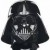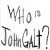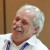# Normal Distribution and Six Sigma

Six Sigma – iSixSigma Forums General Forums Tools & Templates Normal Distribution and Six Sigma

Viewing 9 posts - 1 through 9 (of 9 total)
• Author
Posts
• #54128zelley
Member

1. How do I draw the bell curve to describe six sigma with and without a 1.5 sigma shift ?

2.I check my stats text that show the normal dist table and the Z value ends at 3.0. but for six sigma, the z value is 6. Does anyone has the full table ?

3. I saw a table in
https://controls.engin.umich.edu/wik…_is_6_sigma%3F

# of Standard Deviations % of Total DPMO
1 68.27 690,000
2 95.45 308,537
3 99.73 66,807
4 99.9937 6,210
5 99.99994 233
6 99.9999998 3.4

How is DPMO being derived in the above table?

4. possible to explain normal distribution with six sigma in a simplifed way ?

5. in https://www.isixsigma.com/process-sigma-calculator/process-sigma-calculator-assumptions/
formula:
process Sigma = NORMSINV(1-((Total Defects) / (Total Opportunities))) + 1.5

6. How do i relate the formula “NORMSINV” with the calculations of the formula in normal dist?
ie. z=(y-mean)/(std dev)

Thanks!

0
#193805Darth
Participant

@zellley Thank you so much for sharing your homework assignment with this Forum. It is always our custom to provide students with answers to homework so that it makes the learning experience much easier. Here are the answers:
1. Draw one line at 3 and one at 4.5
2. Full table can be achieved by doubling the 3 sigma table
3. DPMO is derived by taking the table values and dividing by 1.5
4. Yes
5. Is there a question that you are asking?
6. That is a good question and has universal application. How do we all relate with the universe?

Good luck.

0
#193812zellley
Guest

homework? i am NOT a fulltime student getting answers but rather i am an working adult trying to know more about six sigma. i don’t think schools will ask such questions nowadays anyway. the tables and formulae are just thrown to you and then you wonder how all these are being derived (for eg my DPMO question).

2. Full table can be achieved by doubling the 3 sigma table = i don’t understand.
please illustrate

3. DPMO is derived by taking the table values and dividing by 1.5
= what table values ?
If DPMO is 690,000 and you mentioned value/1.5 =690,000, so value =1035000.
where can i find 1035000 ?

4. Yes = so how would you explain in a simplified way to someone who doesn’t know normal distribution or someone who knows but would like to understand how normal distribution relates to 6 sigma ?

5. Is there a question that you are asking?= no. its numbering error.number 5 is actually number 6.

6. That is a good question and has universal application. How do we all relate with the universe? = what i meant is formula NORMSINV , what does it mean ?
i know the calculation z=(y-mean)/(std dev) but what does NORMSINV means?

i checked on excel formula explanation> http://office.microsoft.com/en-us/excel-help/normsinv-HP005209195.aspx,and basically in short NORMSINV just gives the value of Z with the given probability
“(1-((Total Defects) / (Total Opportunities)))”
is my interpretation right ?

Thanks.

0
#193813zellley
Guest

so does it mean that those DPMO figures are derived by some experts who has research and then come up with this “standard” ??

0
#193815zellley
Guest

No one has answers to my question ??

0
#193816MBBinWI
Participant

@zellley – we have real lives. We’re not here merely to service you. We do this as a pasttime or for giggles.
What you need is a personal teacher/mentor. Why would you throw out questions on a forum and just accept the answers?
btw – we mostly appreciate those who do their own due diligence (have you researched the answer yourself, or just looking for a handout from those of us who have already done the hard work and really learned this stuff?) and attempt answering the question, posit a query with their rationale (if they are unsure), and politely enquire for help.
Why don’t you do some reading on this site first. Many of your answers will be found. The site owner has even made this easy for you by putting a “new to six sigma” button near the top of every page on the right side.

0
#193817MBBinWI
Participant

@Darth – #2: Weren’t you required to memorize the table? I was. Zeesh, and to think that I used to look up to you…

0
#193822sriram
Member

Consider this example:
Suppose a project has 125 total defects and consumed 50000 hours, then we have the defect density(say) = 125/50000 = .0025. Let’s now calculate the Yield for this process measurement, Y = e^(-0.0025) = 99.75% and Prob(defects) = 1-Yield = 100%-99.75% = 0.25% defects
Converting this into DPMO values, we get .0025*1000000 = 2497 defects per million opportunities.
So, for 2497dpmo, the sigma level = 4.6 sigma(approx)

0
#193830Mike Carnell
Participant

@zellley You have had two really good resources try to help you out @MBBinWI and @Darth. We don’t normally tell Darth he has done a good job because it goes to his head. It isn’t that the inside of his head is overly crowded but he does become difficult to deal with once he thinks he has added value.

Let me make a suggestion that may help you. There is a site that does a really good job of teaching some of the more fundimental topics around things like the normal distribution.

Check out http://www.khanacademy.org/math/statistics/v/normal-distribution-excel-exercise. There is a drop down menu that has a lot of different topics around statistics. This seems to be pretty well done. I hope it helps.

Just my opinion.

0
Viewing 9 posts - 1 through 9 (of 9 total)

You must be logged in to reply to this topic.